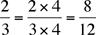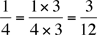Name: barbara Who is asking: Student Level of the question: Middle Question: I know that to subtract 1/4 from 2/3 I must find a common denom. Now the 2/3 becomes 8/12.....i understand the 12, but where does the 8 come from? Hi Barbara, The common denominator 12 you found from the denominators 4 and 3. Next you have to replace both of the fractions 1/4 and 2/3 by equivalent fractions with the denominator. Looking at the fraction 2/3, to get a denominator of 12 you multiply the denominator 3 by 4. Thus to obtain an equivalent fraction with the denominator 12 you need to multiply the numerator by 4 also. That isFor the other fraction 1/4, to get a denominator of 12 you need to multiply of 4 by 3 and hence you need to multiply the numerator by 3 to obtain an equivalent fraction.I hope this helps, Penny